Function Repository Resource:

# VerticalJumpOnAstronomicalBodies

Compute vertical jump heights from the surfaces of astronomical bodies

Contributed by: Jeff Bryant
 ResourceFunction["VerticalJumpOnAstronomicalBodies"][entity] gives the vertical height of a jump from the surface of the astronomical body entity. ResourceFunction["VerticalJumpOnAstronomicalBodies"][entity,vel] gives the vertical height assuming the initial velocity to be vel. ResourceFunction["VerticalJumpOnAstronomicalBodies"][entity,hgt] gives the vertical height assuming that hgt would be the height of the jump on Earth.

## Details and Options

ResourceFunction["VerticalJumpOnAstronomicalBodies"] assumes a constant gravitational acceleration and no air resistance.
ResourceFunction["VerticalJumpOnAstronomicalBodies"] applies only to those bodies with a known acceleration due to gravity.
ResourceFunction["VerticalJumpOnAstronomicalBodies"] applies to astronomical objects that are one of planet, moon, minor planet, comet, exoplanet or star.
If only the astronomical body is provided, the computation assumes an initial jumping speed of about 2.8 m/s.
If quantity is in units of speed, then the the result is computed assuming that initial jumping speed.
If quantity is a number, then the result is computed assuming that initial speed in m/s.
If quantity is in units of length, then the result is computed assuming that equivalent jumping height on Earth.

## Examples

### Basic Examples (2)

Determine the typical jumping height of a human on various astronomical bodies:

 In:=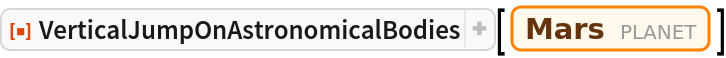Out=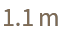In:=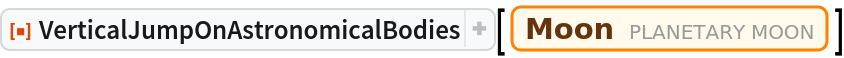Out=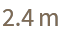Determine the typical jumping height of a human on multiple astronomical bodies:

 In:=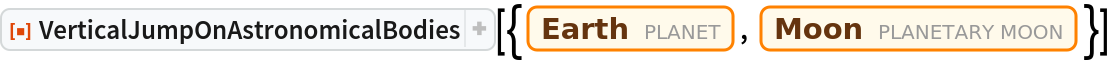Out=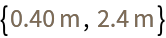### Scope (4)

Vertical jump height can also be computed on minor planets, comets, exoplanets and stars:

 In:=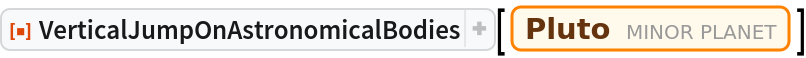Out=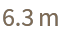In:=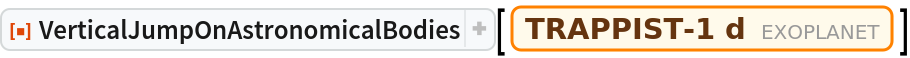Out=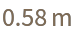In:=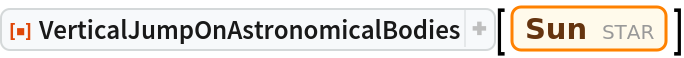Out=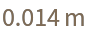The default value for initial jump velocity is 2.8 m/s:

 In:=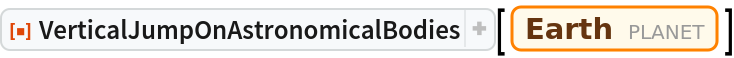Out=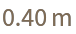The initial velocity can be changed to result in a higher or lower jump on the specified body:

 In:=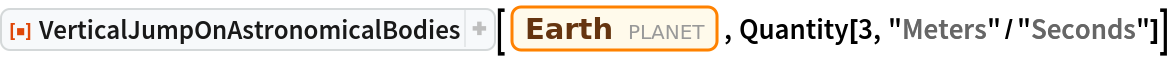Out=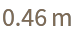If the specified quantity is in height units, computations assume that jump height on Earth and results are scaled accordingly:

 In:=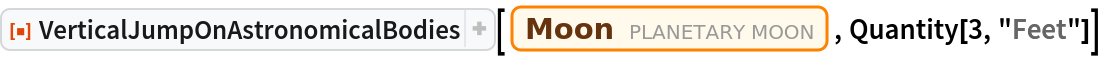Out=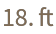In:=Out=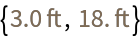Some astronomical bodies have very low gravity, so the height of a jump can be extreme:

 In:=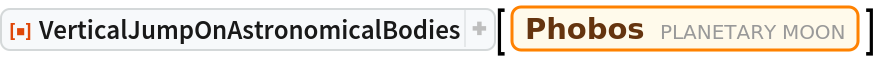Out=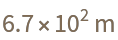In fact, the escape velocity of such bodies can be relatively low:

 In:=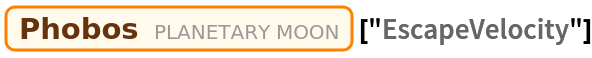Out=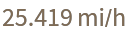If you specify an initial velocity that is higher than the escape velocity of the body, then the vertical height of a jump is infinite:

 In:=Out=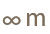## Version History

• 1.0.0 – 30 January 2020